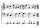Equation algebraogram

Solve the equation:

oco + ivo = cita

How much has the task of solutions?

Result

n =  18

Solution:Leave us a comment of this math problem and its solution (i.e. if it is still somewhat unclear...):Be the first to comment!To solve this verbal math problem are needed these knowledge from mathematics:

Do you have a linear equation or system of equations and looking for its solution? Or do you have quadratic equation?

Next similar math problems:

1. Eq1Solve equation: 4(a-3)=3(2a-5)
2. Simple equation 8Solve the following equation: 36=-(1+7x)-6(-7-x)
3. Seven timesWhich number seven times is just as higher as 27, how much is smaller than 29?
4. Apples 3Julka has 5 apples more than Hugo and four apples less than Annie. Hugo has 17 apples. How many apples has Julka and how Annie?
5. PopsiclesFrancis went to buy ice lollies. If he buy 8 popsicles he missed 4 USD. When he buy 7 popsicles, got back 1 USD. How many USD was a popsicle?
6. I thinkI think a number. When I multiply it by five, and after that I subtract 477, I get the same number as if I multiplied it twice. What number do I think?
7. Number unknownAdela thought the two-digit number, she added it to its ten times and got 407. What number does she think?
8. Forest nurseryIn the forest nursery after winter, they found that 1/10 stems died out of them. For them, they land 193 new spruces. How many spruces are in the forest nursery?
9. If-then equationIf 5x - 17 = -x + 7, then x =
10. Six te 2If 3t-7=5t, then 6t=
11. Number of songsWrite an expression for the number of songs they need for this show. Evan and Peter have a radio show that has 2 parts. They need 4 fewer than 11 songs in the first part. In the second part, they need 5 fewer than 3 times the number of songs in the first p
12. CagesHonza had three cages (black, silver, gold) and three animals (guinea pig, rat and puppy). There was one animal in each cage. The golden cage stood to the left of the black cage. The silver cage stood on the right of the guinea pig cage. The rat was in the
13. Foot in busIt was 102 people on the bus. 28 girls had two dogs. A 11 girls had one dog. At the next stop seceded 5 dogs (even with their owners). They got two boys together with three dogs. The bus drove one driver. How many foot were in bus?
14. Unknown number 16My number's tens is three times more then ones My number's ones is twice the number of thousands and my number's hundreds is half the number of of tens. I have two ones. which number am I?
15. Addition of Roman numbersAdded together and write as decimal number: LXVII + MLXIV
16. Write decimalsWrite in the decimal system the short and advanced form of these numbers: a) four thousand seventy-nine b) five hundred and one thousand six hundred and ten c) nine million twenty-six
17. Roman numeralsWrite numbers written in Roman numerals as decimal.